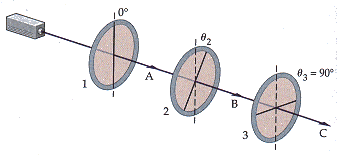# Three polarizers are arranged as shown in the figure

## Homework Statement

Three polarizers are arranged as shown in the figure. If the incident beam of light is unpolarized, and has an intensity of 1.60 W/m2, find the transmitted intensity for each of the following cases.(a) θ2 = 29.6° and θ3 = 44.0°
(b) θ2 = 44.0° and θ3 = 29.6°

I= 1/2I0
I= I0cos2θ

## The Attempt at a Solution

So what I did what for the first area i used
I= 1/2I0 and I got .8
then I2= .8cos2(29.6)= .6048
then for I3= .6048cos2(44.0) =.2918

then i did the same for part b only switched the theta as needed.

Both of the answers are wrong. Ive been trying to think of why this could be wrong. I have tried many different ways, but this seems to be the one that makes most sense to me. If i could get any help that would be nice. Thanks in advance.

Redbelly98
Staff Emeritus
Homework Helper

## The Attempt at a Solution

So what I did what for the first area i used
I= 1/2I0 and I got .8
then I2= .8cos2(29.6)= .6048
then for I3= .6048cos2(44.0) =.2918
For that last step -- you need to use the angle between the 3rd polarizer's axis and the polarization of the light incident on that polarizer.